Custom SearchSOLUTION: Substituting into equation (6.7), we have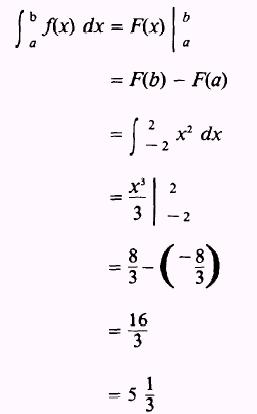The area above a curve and below the X axis, as shown in figure 6-11, will, through integration, furŁnish a negatvie answer.Figure 6-11.-Area above a curve. If the graph of y = f(x), between x = a and x = b, has portions above and portions below the X axis, as shown in figure 6-12, thenis the sum of the absolute values of the positive areas above the X axis and the negative areas below the X axis, such that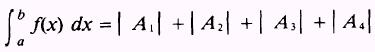where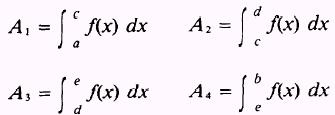Figure 6-12.-Areas above and below a curve.   EXAMPLE: Find the areas between the curve y=x and the X axis bounded by the lines x= -2 and x=2 as shown in figure 6-13.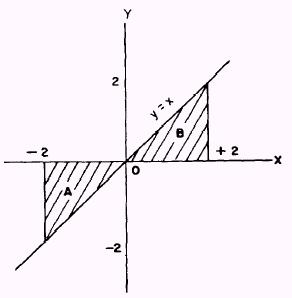Figure 6-13.-Negative and positive value areas. SOLUTION. These areas must therefore, we write be computed separately;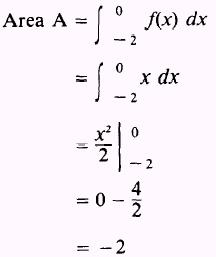and the absolute value of - 2 is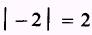Then,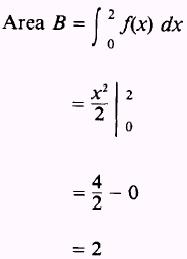Adding the two areas,, we find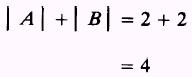NOTE: If the function is integrated from - 2 to 2, the followŁing INCORRECT result will occur: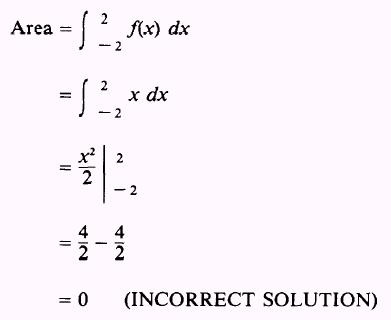This is obviously not the area shown in figure 6-13. Such an exŁample emphasizes the value of making a commonsense check on every solution. A sketch of the function will aid this comŁmonsense judgement. EXAMPLE: Find the total area bounded by the curve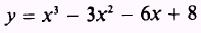the X axis, and the lines x= -2 and x=4 as shown in figure 6-14. SOLUTION. The area desired is both above and below the X axis; therefore, we need to find the areas separately and then add them together using their absolute values.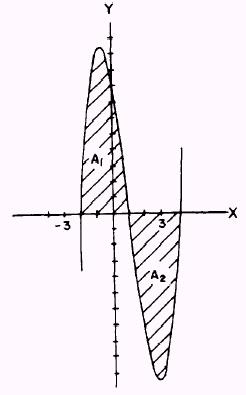Figure 6-14.-Positive and negative value areas. Therefore,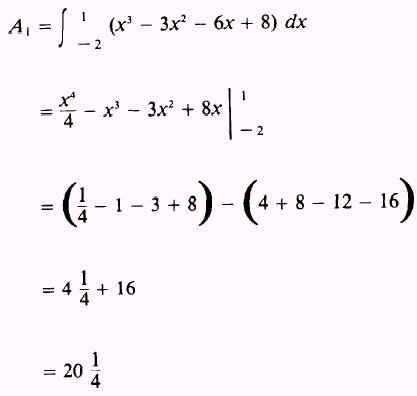and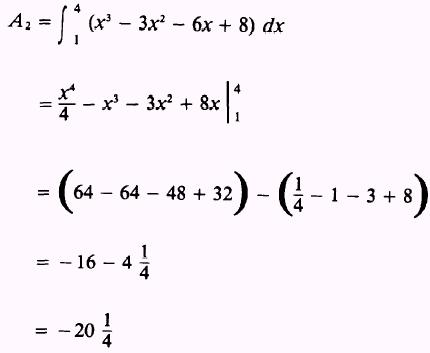Then, the total area is